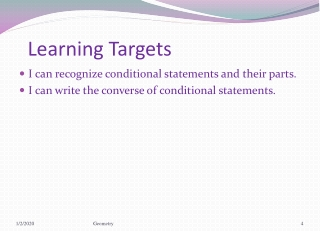DownloadDownload PresentationLearning Targets

# Learning Targets

Download Presentation## Learning Targets

- - - - - - - - - - - - - - - - - - - - - - - - - - - E N D - - - - - - - - - - - - - - - - - - - - - - - - - - -
##### Presentation Transcript

1. Learning Targets • I can recognize conditional statements and their parts. • I can write the converse of conditional statements. Geometry

2. Conditional Statement • A conditional statement has two parts, a hypothesis and a conclusion. • When conditional statements are written in if-then form, the part after the “if” is the hypothesis, and the part after the “then” is the conclusion. • p → q Geometry

3. Example 1: State the hypothesis and conclusion. • If you are 13 years old, then you are a teenager. • Hypothesis: • You are 13 years old • Conclusion: • You are a teenager Geometry

4. Example 1: Rewrite in the if-then form • All mammals breathe oxygen • If an animal is a mammal, then it breathes oxygen. • A number divisible by 9 is also divisible by 3 • If a number s divisible by 9, then it is divisible by 3. Geometry

5. Negation • The negative of the statement • Example: Write the negative of the statement • A is acute • A is not acute • ~p represents “not p” or the negation of p Geometry

6. Converse, Inverse and Contrapositive • Converse • The converse of a conditional is formed by switching the hypothesis and the conclusion. • The converse of p → q is q → p • Inverse • Negate the hypothesis and the conclusion • The inverse of p → q, is ~p → ~q • Contrapositive • Negate the hypothesis and the conclusion of the converse • The contrapositive of p → q, is ~q → ~p. Geometry

7. Example • Write the (a) inverse, (b) converse, and (c) contrapositive of the statement. • If two angles are vertical, then the angles are congruent. • (a) Inverse: If 2 angles are not vertical, then they are not congruent. • (b) Converse: If 2 angles are congruent, then they are vertical. • (c) Contrapositive: If 2 angles are not congruent, then they are not vertical. Geometry

8. Equivalent Statements • When 2 statements are both true or both false • A conditional statement is equivalent to its contrapositive. • The inverse and the converse of any conditional are equivalent. Geometry

9. Geometry